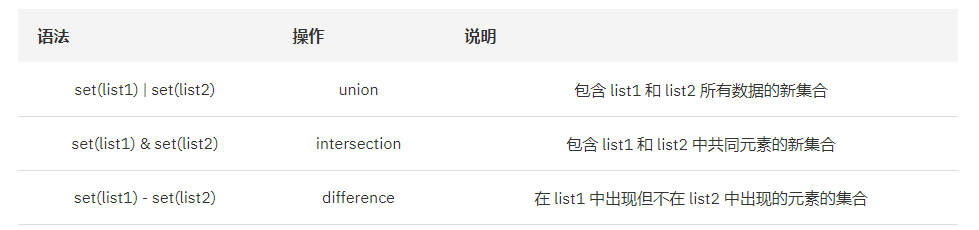# Python 代码性能优化

hresh 422## 前言

### Python 的优劣势

Python 的优势

1. 简单，优雅，易学
2. 可移植性——由于 Python 开源的本质，它已经被移植到了大多数平台下面，例如：Windows、MacOS、Linux、Andorid、iOS 等等。
3. 可扩展性——如果你需要你的一段关键代码运行得更快或者希望某些算法不公开，你可以把你的部分程序用 C 或 C++ 编写，然后在你的 Python 程序中使用它们。
4. 可嵌入性——你可以把 Python 嵌入你的 C/C++ 程序，从而向你的程序用户提供脚本功能。
5. 丰富的第三方库 Python 具有本身有丰富而且强大的库，而且由于 Python 的开源特性，第三方库也非常多，例如：在 web 开发、爬虫、科学计算等等

Python 的劣势

1. 运行速度慢。
2. 既是优点也是缺点，Python 的开源性使得 Python 语言不能加密，但是目前国内市场纯粹靠编写软件卖给客户的越来越少，网站和移动应用不需要给客户源代码，所以这个问题就是问题了。

### Python 为什么性能差

1、Python 是动态强类型语言

2、Python 是解释性语言

3、Python 中一切皆对象

Python 中一切都是对象，“everything is object”，包括类，类的实例，数字，模块。每个对象都需要维护引用计数，增加了额外的工作。

4、Python 中的 GIL

GIL 是 Python 最为诟病的一点，因为 GIL，Python 中的多线程并不能真正的并发。如果是在 IO BOUND 的业务场景，这个问题并不大，但是在 CPU BOUND 的场景，这就很致命了。一般都是使用多进程（pre fork），或者在加上协程。

5、垃圾回收

## Python 代码优化常见技巧

O(1) -> O(lg n) -> O(n lg n) -> O(n^2) -> O(n^3) -> O(n^k) -> O(k^n) -> O(n!)

1、使用 dict 或 set 查找元素

Python 字典和集合中使用了 hash table，因此查找操作的复杂度为 O(1)，而 list 实际是个数组，在 list 中，查找需要遍历整个 list，其复杂度为 O(n)，因此对成员的查找访问等操作字典要比 list 更快。

from cProfile import Profile

def search():
s_list = ['a', 'b', 'is', 'python', 'jason', 'hello', 'hill', 'with', 'phone', 'test',
'dfdf', 'apple', 'pddf', 'ind', 'basic', 'none', 'baecr', 'var', 'bana', 'dd', 'wrd']

#s_list = dict.fromkeys(s_list,True)
#s_list = set(s_list)
results = []

for i in range(1000000):
for s in ['is','hat','new','list','old','.']:
if s not in s_list:
results.append(s)

if __name__ == '__main__':
prof = Profile()
prof.runcall(search)
prof.print_stats()


2、使用 set 求交集、并集和差

set 的 union， intersection，difference 操作要比 list 的迭代要快。因此如果涉及到求 list 交集，并集或者差的问题可以转换为 set 来操作。from cProfile import Profile

def get_intersection():
list_a = list(range(1000))
list_b = list(range(1000))

for i in range(1000):
# intersection = []
# for a in list_a:
#     if a in list_b:
#             intersection.append(a)
intersection = list(set(list_a) & set(list_b))

def get_union():
list_a = list(range(1000))
list_b = list(range(1000))

for i in range(1000):
# union = []
# union.extend(list_a)
# for b in list_b:
#     if b not in list_a:
#         union.append(b)
union = list(set(list_a) | set(list_b))

def get_difference():
list_a = list(range(1000))
list_b = list(range(1000))

for i in range(1000):
# difference = []
# for a in list_a:
#     if a not in list_b:
#         difference.append(a)
difference = list(set(list_a) - set(list_b))

def list_operation():
get_intersection()
get_union()
get_difference()

if __name__ == "__main__":
prof = Profile()
prof.runcall(list_operation)
prof.print_stats()


3、优化循环

from cProfile import Profile

def loop_work():
list_a = list(range(100))
list_b = list(range(100))

len_a = len(list_a)
len_b = len(list_b)

#循环优化前
for i in range(10000):
for i in range(len(list_a)):
for j in range(len(list_b)):
x = list_a[i] + list_b[j]
#循环优化后
# for i in range(10000):
#     for i in range(len_a):
#         x = list_a[i]
#         for j in range(len_b):
#             x += list_b[j]

if __name__ == '__main__':
prof = Profile()
prof.runcall(loop_work)
prof.print_stats()


4、优化包含多个判断表达式的顺序

from cProfile import Profile

def or_work():
nums = range(2000)

for i in range(10000):
#优化前
new_nums = [x for x in nums if 10 < x < 20 or 1000 < x < 2000]
# 优化后
# new_nums = [x for x in nums if 1000 < x < 2000 or 100 < x < 20]

def and_work():
nums = range(2000)

for i in range(10000):
# 优化前
new_nums = [x for x in nums if x % 2 == 0 and x > 1900]
# 优化后
# new_nums = [x for x in nums if x > 1900 and x % 2 == 0]

def work():
or_work()
and_work()

if __name__ == '__main__':
prof = Profile()
prof.runcall(work)
prof.print_stats()


5、使用 join 合并迭代器中的字符串

6、合理使用生成器（generator）

7、使用局部变量，避免"global" 关键字。python 访问局部变量会比全局变量要快得多，因 此可以利用这一特性提升性能。

8、使用 if is 进行判断

if done is not None 比语句 if done != None 更快

x = None

for i in range(1000000):
#优化前
# if x != None:
#     key = "word"
#优化后
if x is not None:
key = "word"


9、使用级联比较 "x < y < z" 而不是 "x < y and y < z"。

10、while 1 要比 while True 更快（当然后者的可读性更好）。

11、不借助中间变量交换两个变量的值

## Python 优化窍门

Python 是一种非常酷的语言，因为你可以用如此少的代码在如此短的时间内完成它。不仅如此，它还轻松支持许多任务，例如多处理。

Python 批评者有时声称 Python 很慢。但它不一定是这样：尝试这六个技巧来加速你的 Python 应用程序。

1、依靠关键代码的外部包

Python 简化了许多编程任务，但是对于一些时间敏感的任务，它的表现经常不尽人意。使用 C/C++ 或机器语言的外部功能包处理时间敏感任务，可以有效提高应用的运行效率。这些功能包往往依附于特定的平台，因此你要根据自己所用的平台选择合适的功能包。简而言之，这个窍门要你牺牲应用的可移植性以换取只有通过对底层主机的直接编程才能获得的运行效率。以下是一些你可以选择用来提升效率的功能包：

2、在排序时使用键

Python 含有许多古老的排序规则，这些规则在你创建定制的排序方法时会占用很多时间，而这些排序方法运行时也会拖延程序实际的运行速度。最佳的排序方法其实是尽可能多地使用键和内置的 sort()方法。譬如，拿下面的代码来说：

import operator
somelist = [(1, 5, 8), (6, 2, 4), (9, 7, 5)]
somelist.sort(key=operator.itemgetter(0))
somelist
#Output = [(1, 5, 8), (6, 2, 4), (9, 7, 5)]
somelist.sort(key=operator.itemgetter(1))
somelist
#Output = [(6, 2, 4), (1, 5, 8), (9, 7, 5)]
somelist.sort(key=operator.itemgetter(2))
somelist
#Output = [(6, 2, 4), (9, 7, 5), (1, 5, 8)],


3、优化循环

lowerlist = ['this', 'is', 'lowercase']
upper = str.upper
upperlist = []
append = upperlist.append
for word in lowerlist:
append(upper(word))
print(upperlist)
#Output = ['THIS', 'IS', 'LOWERCASE']


（注意：优化循环的方法还有很多，这只是其中之一。比如，很多程序员会认为，列表推导式是提高循环速度的最佳方法。关键在于，优化循环方案是提高应用程序运行速度的上佳选择。）

4、使用较新的版本

5、尝试多种编码方法

n = 16
myDict = {}
for i in range(0, n):
char = 'abcd'[i%4]
if char not in myDict:
myDict[char] = 0
myDict[char] += 1
print(myDict)


    n = 16
myDict = {}
for i in range(0, n):
char = 'abcd'[i%4]
try:
myDict[char] += 1
except KeyError:
myDict[char] = 1
print(myDict)


6、交叉编译你的应用

Nuitka 是一款有趣的交叉编译器，能将你的 Python 代码转化成 C++代码。这样，你就可以在 native 模式下执行自己的应用，而无需依赖于解释器程序。你会发现自己的应用运行效率有了较大的提高，但是这会因平台和任务的差异而有所不同。